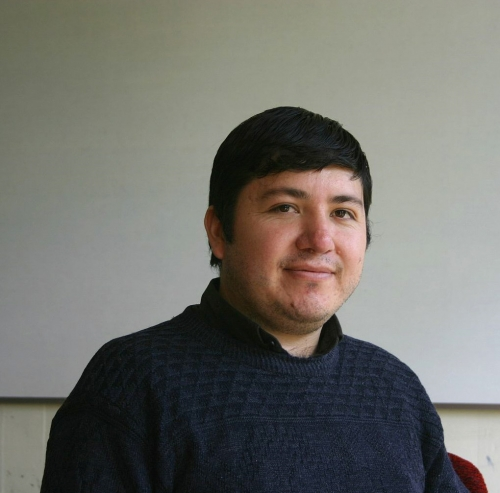﻿﻿ CI²MA - Publications | Graduate Thesis

# Graduate Thesis of Anibal CoronelProgramPhD in Applied Sciences with mention in Mathematical Engineering, Universidad de Concepción
Enrollment Year1998
Senior Year2004
Thesis TitleStudy of a Reverse Problem for a Degenerate Parabolic Equation with Applications to Sedimentation Theory

#### Thesis Summary:

In this thesis we study an inverse problem for a strongly degenerate parabolic equation modelling the separation of a mixture of solid and fluid by sedimentation. The inverse problem (IP) consists in the determination of coefficients of the differential equation governing the processes from concentration measurements, which is the unknown of the model or direct problem. We formulate the IP as a minimization problem for an appropriate cost function which optimize the difference of the solution of the model with the observations. We analyze the IP for two cases of interest: the sedimentation under the acceleration of gravity (sedimentation) and the sedimentation by the influence of centripetal forces (centrifugation). In both cases, we prove a continuous dependence of entropy solution with respect to the coefficients, which implies the existence of solutions for IP. We obtain the stationary points of the cost function by a steepest descent method where the gradient is formally calculated by a Lagrangian formulation which permits the introduction of an adjoint state consisting in a linear backward boundary value problem for a strongly degenerate parabolic equation with discontinuous coefficients. Following a similar computation to the formal deduction of the gradient, we obtain an efficient computation of the exact gradient for the discrete model. This method is used to identify the parameters of the flux density function and diffusion coefficient in the constitutive relations obtained by the modelling assumptions.

Thesis Director(s) Mauricio Sepúlveda
Thesis Project Approval Date2000, March 03
Thesis Defense Date2004, September 30
Professional MonitoringOctober 2004 to date: Assistant Professor, Departamento de Matematicas, Universidad del Bio-Bio (sede Chillan), Chillan.
PDF ThesisDownload Thesis PDF#### ISI Publications from the Thesis

Raimund BüRGER, Aníbal CORONEL, Mauricio SEPúLVEDA: On an upwind difference scheme for strongly degenerate parabolic equations modelling the settling of suspensions in centrifuges and non-cylindrical vessels. Applied Numerical Mathematics, vol. 56, Nos. 10-11 (2006), 1397-1417

Raimund BüRGER, Aníbal CORONEL, Mauricio SEPúLVEDA: A semi-implicit monotone difference scheme for an initial- boundary value problem of a strongly degenerate parabolic equation modelling sedimentation-consolidation processes. Mathematics of Computation, vol. 75, No. 243, pp. 91-112, (2006)

Stefan BERRES, Raimund BüRGER, Aníbal CORONEL, Mauricio SEPúLVEDA: Numerical identification of parameters for a flocculated suspension from concentration measurements during batch centrifugation. Chemical Engineering Journal, vol. 111, 2-3, pp. 91-103 (2005)

Stefan BERRES, Raimund BüRGER, Aníbal CORONEL, Mauricio SEPúLVEDA: Numerical identification of parameters for a strongly degenerate convection–diffusion problem modelling centrifugation of flocculated suspensions. Applied Numerical Mathematics, vol. 52, 4, pp. 311-337, (2005)

Aníbal CORONEL, Francois JAMES, Mauricio SEPúLVEDA: Numerical identification of parameters for a model of sedimentation processes. Inverse Problems, vol. 19, 4, pp. 951-972, (2003)

#### No-ISI Publications from the Thesis

Raimund BüRGER, Aníbal CORONEL, Mauricio SEPúLVEDA: A numerical descent method for an inverse problem of a scalar conservation law modelling sedimentation. Numerical Mathematics and Advanced Applications, pp. 225-232, Springer Verlag,(2008)

<< Back to list of Graduate Thesis.

 CI²MA, CENTER FOR RESEARCH IN MATHEMATICAL ENGINEERING, UNIVERSIDAD DE CONCEPCIÓN - MAILBOX 160-C, CONCEPCIÓN, CHILE, PHONE: +56-41-2661324